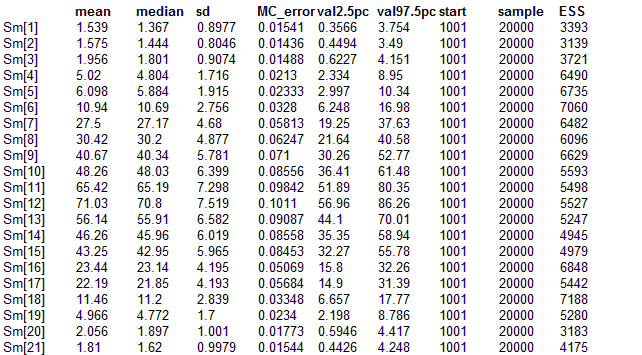#### Pig Weight Gain

Histogram smoothing adapted from Example 5.9 from Congdon (2001), p 180. The model illustrates a structured precision matrix for a multivariate normal prior on a multinomial logistic regression model. The model can also be formulated in terms of a structured covariance matrix (see the commented out section of the model) which has a form with elements that decay exponentialy with absolute value of difference in index.

model{
y[1:s] ~ dmulti(th[1 : s] , n)
sum.g <- sum(g[])
# smoothed frequencies
for (i in 1 : s) {
Sm[i] <- n * th[i]
g[i] <- exp(gam[i])
th[i] <- g[i] / sum.g
}
# prior on elements of AR Precision Matrix
rho ~ dunif(0, 1)
tau ~ dunif(0.5, 10)
# MVN for logit parameters
gam[1 : s] ~ dmnorm(mu[], T[ , ])
for (j in 1:s) {
mu[j] <- -log(s)
}
# Define Precision Matrix
for (j in 2 : s - 1) {
T[j, j] <- tau * (1 + pow(rho, 2))
}
T[1, 1] <- tau
T[s, s] <- tau
for (j in 1 : s -1 ) {
T[j, j + 1] <- -tau * rho
T[j + 1, j] <- T[j, j + 1]
}
for (i in 1 : s - 1) {
for (j in 2 + i : s) {
T[i, j] <- 0; T[j, i] <- 0
}
}
# Or Could do in terms of covariance, which is simpler to write but slower
#      for (i in 1 : s) {
#         for (j in 1 : s) {
#            cov[i, j] <- pow(rho, abs(i - j)) / tau
#         }
#      }
#      T[1 : s, 1 : s] <- inverse(cov[ , ])
}

##### Data
``` list(y = c(1,1,0,7,5,10,30,30,41,48,66,72,56,46,45,22,24,12,5,0,1), n = 522, s = 21) ```
##### Inits for chain 1
``` list(gam=c(-3,-3,-3,-3,-3,-3,-3,-3,-3,-3,-3,-3,-3,-3,-3,-3,-3,-3,-3,-3,-3))    ```

##### Inits for chain 2
``` list(gam=c(-1.0,-1.0,-1.0,-1.0,-1.0,-1.0,-1.0,-1.0,-1.0,-1.0,-1.0,-1.0,-1.0,-1.0,-1.0,-1.0,-1.0,-1.0,-1.0,-1.0,-1.0))    ```
Results